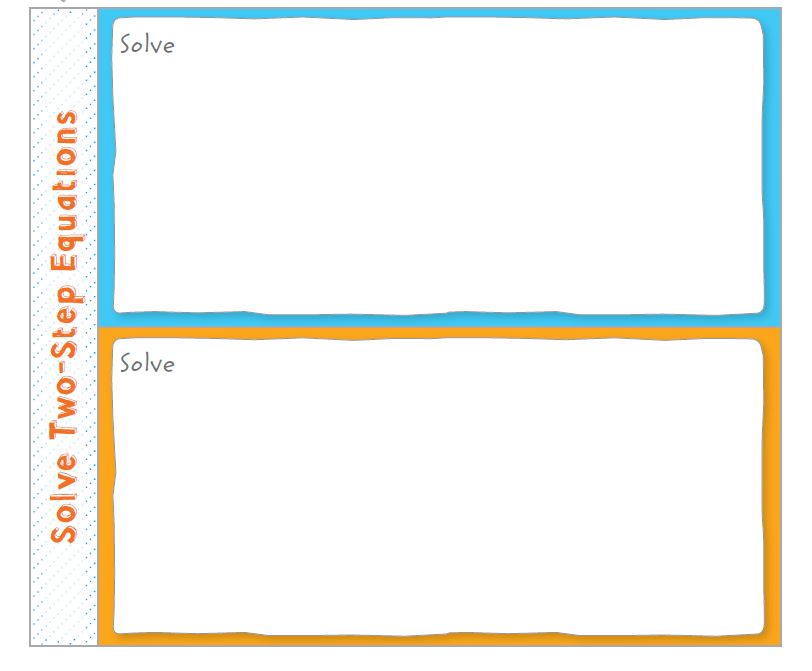Homework Explained - Math Practice 101Dear guest, you are not a registered member. As a guest, you only have read-only access to our books, tests and other practice materials.

As a registered member you can:

Registration is free and doesn't require any type of payment information. Click here to Register.
Go to page:
Chapter 6: Equations and Inequalities; Chapter ReviewUse your Foldable to help review the chapter.Got it?
Match each phrase with the correct term.

• Question 1  1. The value of a variable that makes an equation true a. equivalent equations 2. The numerical factor in a multiplication expression b. equation 3. Equations that have the same solution c. Addition Property of Equality 4. A sentence stating that two quantities are equal d. coefficient e. formula f. solution
•  $$1:$$ $$2:$$ $$3:$$ $$4:$$

Yes, email page to my online tutor. (if you didn't add a tutor yet, you can add one here)